## Solving Equations in Quadratic Form

Any equation in the form ax 2 + bx + c = 0 is said to be in quadratic form. This equation then can be solved by using the quadratic formula, by completing the square, or by factoring if it is factorable.

##### Example 1

Solve x 4 – 13 x 2 + 36 = 0 by (a) factoring and (b) applying the quadratic formula.

1.%

By the zero product rule,2. x 4 – 13 x 2 + 36 = 0

is equivalent toWhen applying the quadratic formula to equations in quadratic form, you are solving for the variable name of the middle term. Thus, in this case,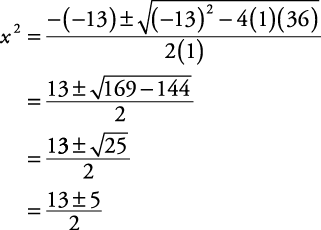Using the square root property,##### Example 2

Solve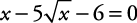by (a) factoring and (b) applying the quadratic formula.

1.In the last step on the right,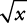must be a nonnegative value; therefore,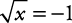has no solutions. The only solution is x = 36.

2.is equivalent to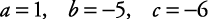When applying the quadratic formula to this quadratic form equation, you are solving for.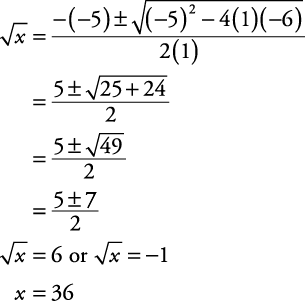There is no solution for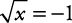. Thus, x = 36 is the only solution.